## Summary and examples

The general equation of a quadratic function is:,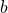and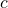are called parameters.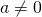, because otherwise it is no quadratic function.

The graph of a quadratic function is a parabola. There are two types, one that opens up and one that opens down, depending on the parameter.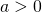: opens up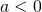: opens down

A parabola intersects the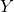-axis in the point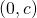. You can easily see this, because for any point on the-axis we have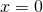. Whenis substituted in the equation we get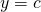.

When a parabola intersects the-axis we can calculate the intersection points in various ways. The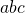-formula, explained in Quadratic equations (abc-formula), always gives a solution. Another way is based on factorizing the equation, see the topic Quadratic equations (factorizing). This is faster, though not always possible.

The intersection points can be calculated by applying the-formula: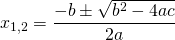In this formula the discrriminant plays an important role: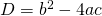We have:

•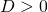: the parabola has two different intersection points with the-axis;
•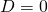: the parabola has two coinciding intersection points with the-axis (usually it is said that the parabola has just one intersection point); and
•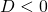: the parabola has no intersection points with the-axis.

A parabola is a symmetric graph and has a symmetry axis, namely the line: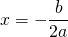Then it is clear that the top of the parabola is on this symmetry axis: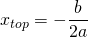This can also be deduced from the-formula. This optimum can also be found by differentiating the function and make the derivative equal to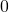: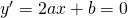#### Example 1

Given the function:1. Determine the intersection point of the graph with the-axis;
2. Does this graph have an intersection point with the-axis, and if so, how many and determine their coordinates.
3. Determine the symmetry axis.

1. Becausein the formula is equal to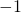, the intersection point with the-axis is equal to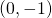.
2. We have to compute the discriminant: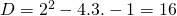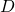is greater thanen thus the graph has two intersection points with the-axis. The-coordinates of these intersection points are: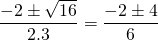so: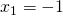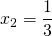and the coordinates are: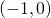and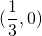3. The symmetry axis can be calculated in two way. First by using the formula above, but also by taking the mean value of the two intersection points, namely:#### Example 2

Given the function: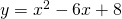1. Determine the intersection point of the graph with the-axis;
2. Does this graph have an intersection point with the-axis, and if so, how many and determine their coordinates.
3. Determine the symmetry axis.

1. Becausein the formula is equal to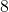, the intersection point with the-axis is equal to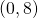.
2. We can compute the discriminant to find out whether there are one or more intersection points, but another way is faster. The equation can be factorized: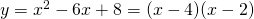and thus we may conclude that the graph has two intersection points with the-axis: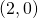en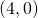.

3. The symmetry axis lies just in the middle of these two points, so the symmetriy-axis is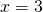.

#### Example 3

For which value of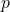does the parabola: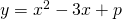have two coinciding intersection points with the-axis.

These intersection points coincide if the discriminant, so: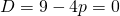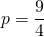0